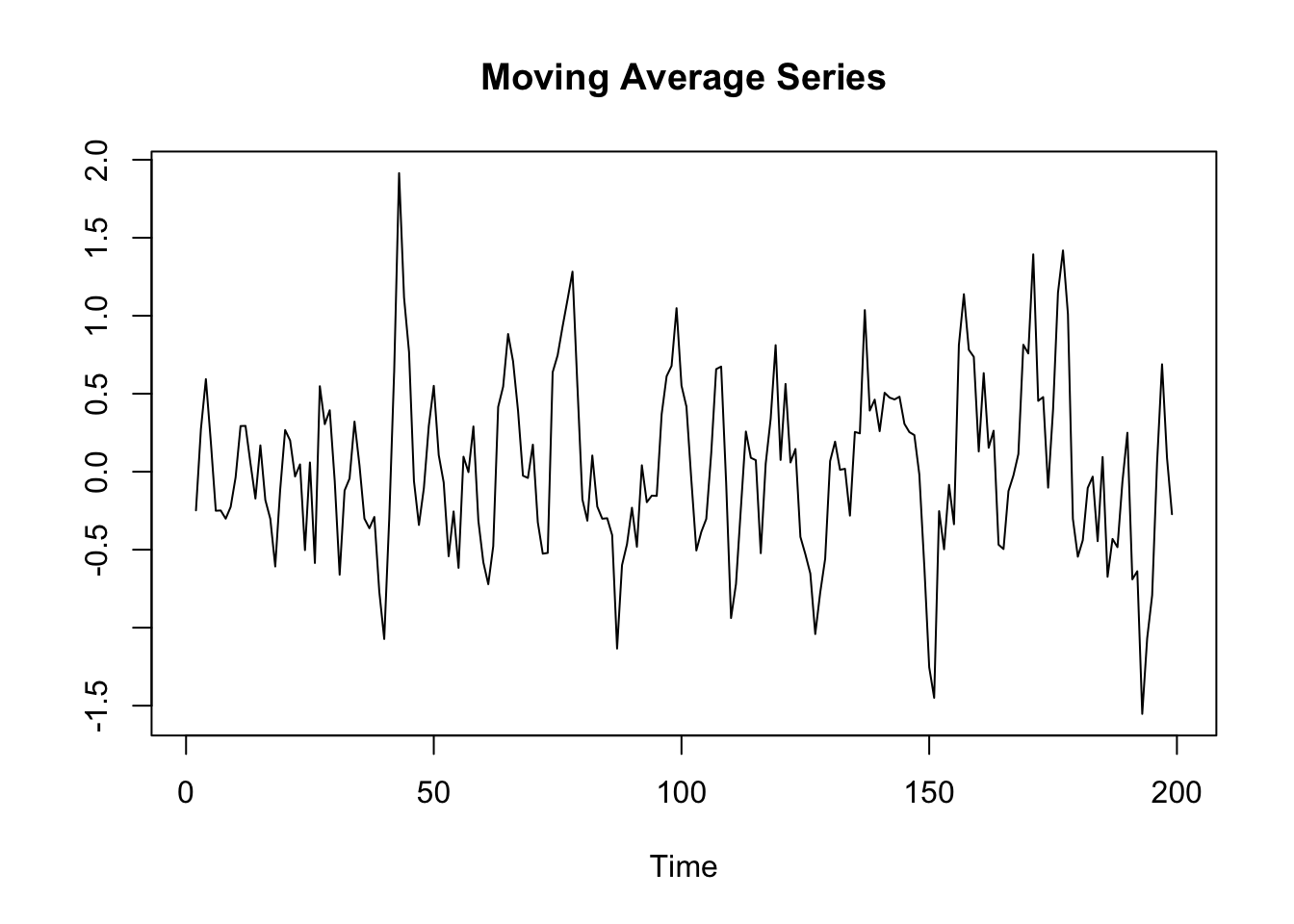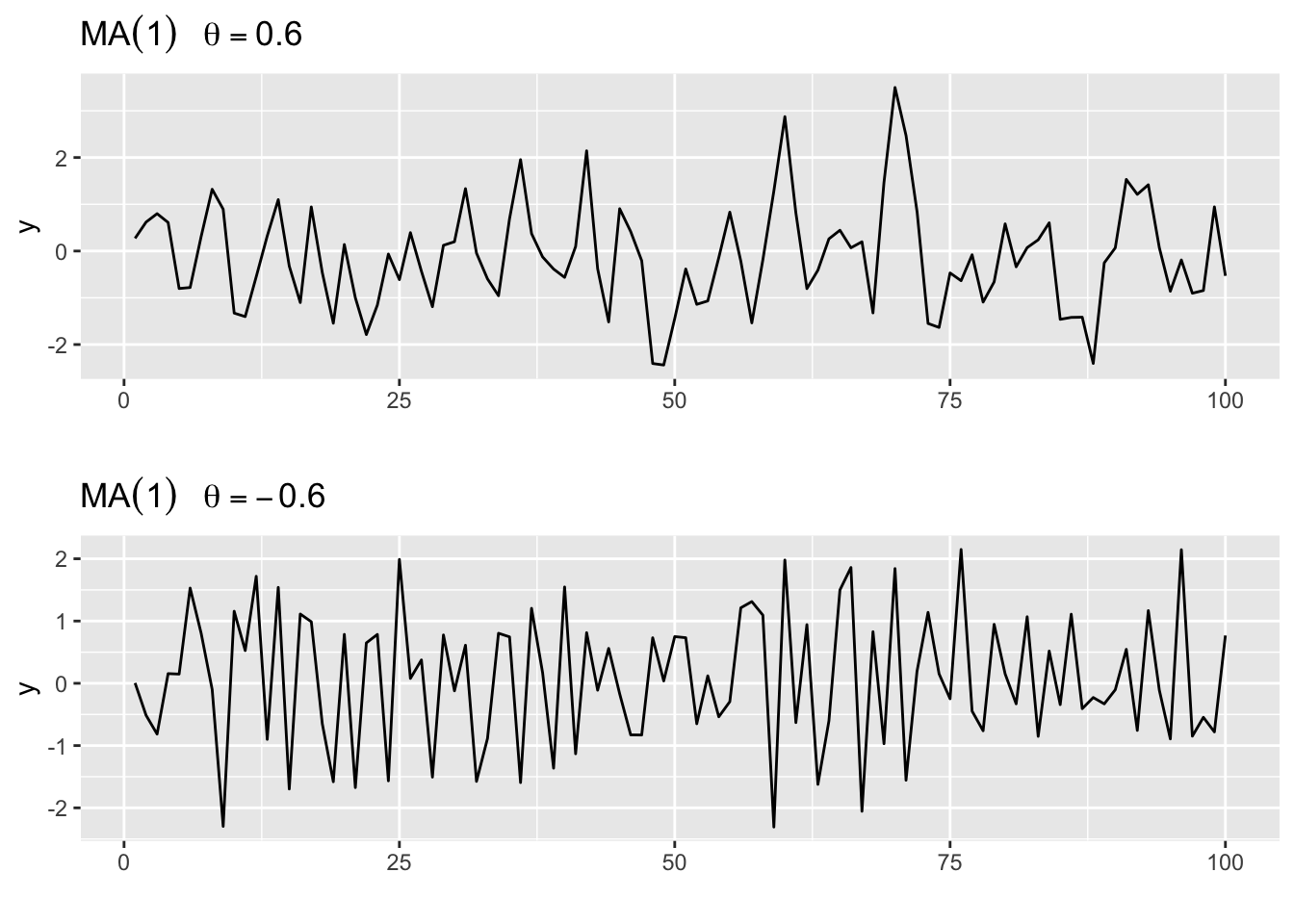The moving average process of order $$q$$ is denoted MA$$(q)$$ and defined by

$y_t = w_t+\theta_1w_{t-1}+...+\theta_qw_{t-q}$ which can be rewritten as

$y_t = \sum_{j=0}^q\theta_jw_{t-j}$

where $$\theta_0 =1$$, $$\theta_1, \theta_2, ..., \theta_q$$ are fixed constants, and $$w_t$$ denotes a random variable with mean $$0$$ and variance $$\sigma^2$$. In the MA model the current value of the series is a weighted sum of past white noise terms. Think of the white noise series as being stochastically uncorrelated information which appears at each time step, and which is combined with other information to provide the observation at $$y_t$$. Hence, moving average models are useful for modeling series that are affected by a variety of unpredictable events where the effect of these events have an immediate effect as well as possible longer term effects.

The moving average process is a stationary process and the serial correlation at lags greater than $$q$$ is zero.

$\gamma(k) = \begin{cases} 0, & \vert k\vert > q \\ \sigma^2\sum_{j=0}^{q-\vert k\vert}\theta_j\theta_{j+k} & \vert k\vert \le q \end{cases}$

In R we can generate a moving average series by applying the filter() function on a white noise series.

set.seed(250)
## white noise series
w <- rnorm(n = 200, mean = 0, sd = 1)
## moving averge
y.ma <- stats::filter(w, rep(1/3,3))
plot.ts(y.ma, main = "Moving Average Series", ylab = "")Another, very convenient way to simulate moving average processes in R is to make use of the arima.sim() function.

We generate two MA(1) series of the form

$y_t = w_t+\theta_1w_{t-1}\text{,}$

where once $$\theta_1 = 0.6$$ and the other time $$\theta_1 = -0.6$$.

ma1.1 <- arima.sim(list(order = c(0,0,1), ma = 0.6), n = 100)
ma1.2 <- arima.sim(list(order = c(0,0,1), ma = -0.6), n = 100)

We plot the two series using the autolplot() function.

library(ggfortify)
library(gridExtra)

p1 <- autoplot(ma1.1,
ylab = "y",
main = (expression(MA(1)~~~theta==0.6)))

p2 <- autoplot(ma1.2,
ylab = "y",
main = (expression(MA(1)~~~theta==-0.6)))

grid.arrange(p1, p2, ncol = 1)When $$\theta = 0.6$$, $$y_t$$ and $$y_{t-1}$$ are positively correlated, and when $$\theta = -0.6$$, $$y_t$$ and $$y_{t-1}$$ are negatively correlated. This correlational structure can be shown by plotting the correlogram.

p1 <-  autoplot(acf(ma1.1, plot = F)) +
ggtitle(expression('Serial Correlation ' *MA(1)~~~theta==0.6))
p2 <- autoplot(acf(ma1.2, plot = F)) +
ggtitle(expression('Serial Correlation ' *MA(1)~~~theta==-0.6))
grid.arrange(p1, p2, ncol = 1)